## Hole Theory and Charge Conjugation

Dirac postulated that the negative energy'' sea was entirely filled with electrons and that an anti-electron would be formed if one of the negative energy'' electrons were elevated to a positive energy state. This would yield a positive energy electron plus a hole in the negative energy'' sea. The hole also has positive energy compared to the vacuum since it is lacking the negative energy present in the vacuum state. Therefore, both the electron and the positron would have positive energy. This describes the process of pair creation.

Similarly, any positive energy electron could make a transition to the now empty negative energy'' state. Energy would be given off, for example by the emission of two photons, and the system would return to the vacuum state. This is the process of pair annihilation.

The tables below compare an electron and a positron with the same momentum and spin. For simplicity, the tables assume the momentum is along the z direction so that we can have spin up and spin down eigenstates. The electron and positron have opposite charge so the standard Electromagnetic currents are in opposite directions. The last row of the table shows the negative energy'' electron state that must be unoccupied to produce the positron state shown in the row above it. A hole in the vacuum naturally produces a positron of opposite charge, momentum, and spin. Because the probability flux for the negative energy'' electron states is in the opposite direction of the momentum, (and the charge and momentum are opposite the positron) the EM current of the positron and of the negative energy'' state are in opposite directions, due the product of three signs. This means the velocities are in the same direction.

 charge mom. Energy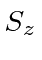spinor spin up, positive energy electron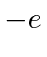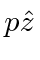spin up, positive energy positron(spin down negative energy'' hole) spin down, negative energy'' electron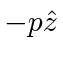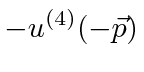We have defined the positron spinorto be the one with positive momentum and spin up. Note that the minus sign on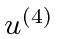is conventional and will come from our future definition of the charge conjugation operator.

Similarly we can make a table starting from a spin down electron.

 charge mom. Energyspinor spin down, positive energy electronspin down, positive energy positron(spin up negative energy'' hole) spin up, negative energy'' electronWe have now also defined the spinor,, for the spin down positron.

Jim Branson 2013-04-22